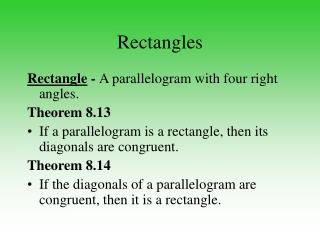# Rectangles - PowerPoint PPT PresentationDownload PresentationRectangles

RectanglesDownload Presentation## Rectangles

- - - - - - - - - - - - - - - - - - - - - - - - - - - E N D - - - - - - - - - - - - - - - - - - - - - - - - - - -
##### Presentation Transcript

1. Rectangles Rectangle - A parallelogram with four right angles. Theorem 8.13 • If a parallelogram is a rectangle, then its diagonals are congruent. Theorem 8.14 • If the diagonals of a parallelogram are congruent, then it is a rectangle.

2. Rectangles Summary of Properties of a Rectangle: • Opposite sides are parallel. • Opposite sides are congruent. • Opposite angles are congruent. • Consecutive angles are supplementary. • Diagonals bisect each other. • All four angles are right angles. • Diagonals are congruent.

3. Quadrilateral EFGH is a rectangle. If and find x. Example 4-1c Answer: 5

4. Example 4-2e Quadrilateral EFGH is a rectangle. a. Findx. b. Find y. Answer: 11 Answer: 7

5. Answer: Since opposite sides are parallel, we know thatRSTU is a parallelogram. We know that . A parallelogram with congruent diagonals is a rectangle. Therefore, the corners are Example 4-3b Max is building a swimming pool in his backyard. He measures the length and width of the pool so that opposite sides are parallel. He also measures the diagonals of the pool to make sure that they are congruent. How does he know that the measure of each corner is 90?

6. Example 4-4f Quadrilateral WXYZ has vertices W(–2, 1), X(–1, 3), Y(3, 1), and Z(2, –1).Determine whether WXYZ is a rectangle using the Distance Formula.